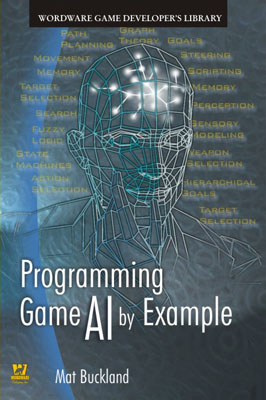Bug Fixes for Programming Game AI by Example

Simple Soccer

There is a minor bug in SoccerPitch::GetRegionFromIndex(). In debug mode it will assert incorrectly for a value of 0 (which is a valid region). here's the change:

 assert ( (idx > 0) && (idx < m_Regions.size()) );

should be replaced with

 assert ( (idx >= 0) && (idx < m_Regions.size()) );

Regarding Luabind and Boost 1.32.0

In Boost 1.32.0 the apply_if metafuction has been renamed to eval_if. This causes errors when trying to compile using the current Luabind release.

Page 183

The SoccerTeam::CanShoot method determines the shot target using x values instead of y values. This is an artifact from when I had the pitch oriented differently (originally it ran vertically instead of horizontally), and is obviously something my not-so-eagle eyes missed when updating the code.

The following snippet shows the changes in green

 //the y value of the shot position should lay somewhere between two //goalposts (taking into consideration the ball diameter) int MinYVal = OpponentsGoal()->LeftPost().y + Pitch()->Ball()->BRadius(); int MaxYVal = OpponentsGoal()->RightPost().y - Pitch()->Ball()->BRadius(); ShotTarget.y = (double)RandInt(MinYVal, MaxYVal);

The updated source and/or executable can be downloaded from the Wordware website (URL given in book, page xxi). You'll find a lot more goals get scored now!

SparseGraph.h

The SparseGraph::isNodePresent method has a bug since the value of nd must be assured to be valid before the test can be made for a valid index. Substitute the line below shown in red for the one show in green

 template bool SparseGraph::isNodePresent(int nd)const {   if ( (m_Nodes[nd].Index() == invalid_node_index) || (nd >= m_Nodes.size()))   {     return false;   }     else return true; }

Like this:

 template bool SparseGraph::isNodePresent(int nd)const {   if ( (nd >= m_Nodes.size()) || (m_Nodes[nd].Index() == invalid_node_index))   {     return false;   }     else return true; }

bugs_at_aijunkie.com